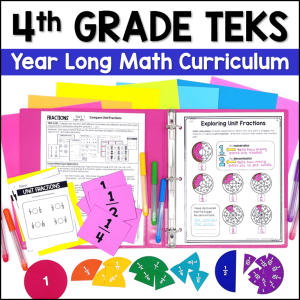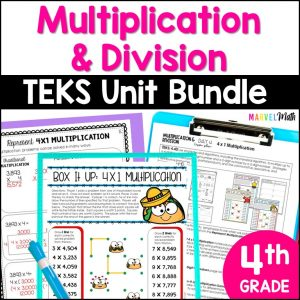Save 10% on your first order with code EXTRA10

# 4th Grade Multiplication & Division STAAR Review Task Cards – Printable

\$4.00187.25\$30.99
SKU: 4119020 Categories: ,

## Description

This set includes 24 STAAR Prep Task Cards to help students practice multiplication and division in word problems, with arrays and the area model, and with equations based on the properties of multiplication.

Both color and blackline cards are included.

I have also included a color-coded diagnostic key to help you make re-teach groups based on the TEKS your students haven’t mastered.

A mini-cover and a mini-key are included. Both are task card sized to help with organization.

4.4B Determine the product of a number and 10 or 100 using properties of operations and place value understandings.

4.4C Represent the product of 2 two-digit numbers using arrays, area models, or equations, including perfect squares through 15 by 15.

4.4D Use strategies and algorithms, including the standard algorithm, to multiply up to a four-digit number by a one-digit number and to multiply a two-digit number by a two-digit number. Strategies may include mental math, partial products, and the commutative, associative, and distributive properties.

4.4E Represent the quotient of up to a four-digit whole number divided by a one-digit whole number using arrays, area models, or equations.

4.4F Use strategies and algorithms, including the standard algorithm, to divide up to a four-digit dividend by a one-digit divisor.

4.4H Solve with fluency one- and two-step problems involving multiplication and division, including interpreting remainders.